# High School Math : Using Basic and Definitional Identities

## Example Questions

### Example Question #1 : Trigonometric Identities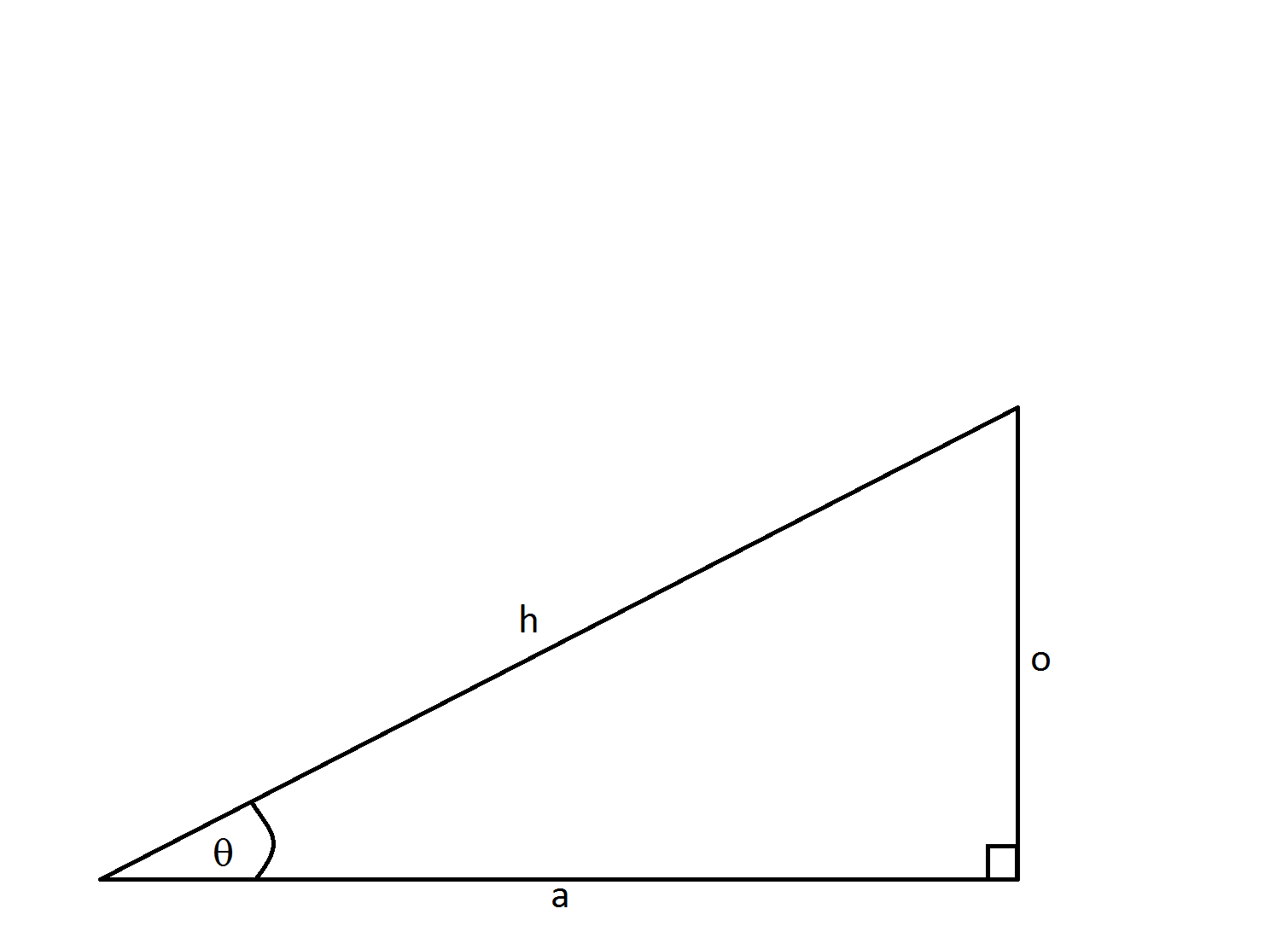What is the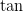of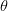?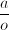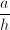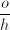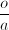Explanation:

When working with basic trigonometric identities, it's easiest to remember the mnemonic: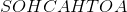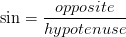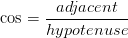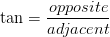When one names the right triangle, the opposite side is opposite to the angle, the adjacent side is next to the angle, and the hypotenuse spans the two legs of the right angle.

### Example Question #2 : Trigonometric Identities

Simplify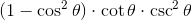.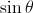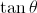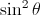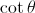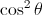Explanation:

Simplifying trionometric expressions or identities often involves a little trial and error, so it's hard to come up with a strategy that works every time. A lot of times you have to try multiple strategies and see which one helps.

Often, if you have any form of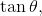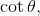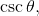or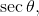in an expression, it helps to rewrite it in terms of sine and cosine. In this problem, we can use the identities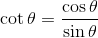and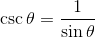.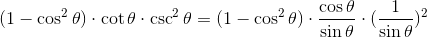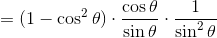.

This doesn't seem to help a whole lot. However, we should recognize that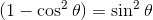because of the Pythagorean identity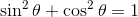.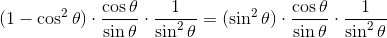We can cancel the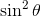terms in the numerator and denominator.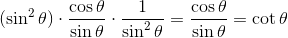.

### Example Question #3 : Trigonometric IdentitiesWhat is the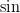of?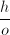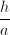Explanation:

When working with basic trigonometric identities, it's easiest to remember the mnemonic:.When one names the right triangle, the opposite side is opposite to the angle, the adjacent side is next to the angle, and the hypotenuse spans the two legs of the right angle.

### Example Question #4 : Trigonometric IdentitiesWhat is the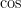of?Explanation:

When working with basic trigonometric identities, it's easiest to remember the mnemonic:.When one names the right triangle, the opposite side is opposite to the angle, the adjacent side is next to the angle, and the hypotenuse spans the two legs of the right angle.